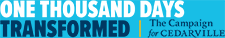# Figuring GPA

To Figure your GPA take the specific letter grade you received in a class and multiply the corresponding number of grade points (see below) by the number of credit hours that class is worth to determine total grade points earned. Repeat this process for each class; then add all grade points earned and divide by the total number of credit hours attempted. The resulting number should equal your GPA.